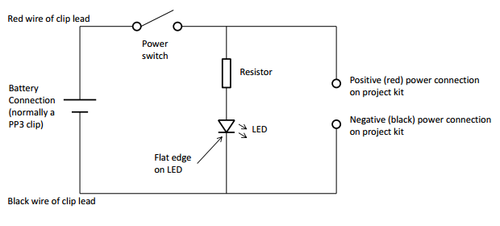The diagram below shows a simple way of adding a power switch and a power LED to a project kit. It is also possible to add either just the power switch or just the power LED.The switch is connected in series with the positive power supply lead. The LED is connected in parallel with the project kit and is placed after the switch (if it were to be placed before the switch it would always be on).Like diodes LEDs drop some voltage across them, this is typically 1.8 volts for a standard LED. If the LED in the above circuit is connected to a 9 Volt supply, there must be a total of 9 Volts dropped across the LED (VLED) and the resistor (VR). If a standard red the LED is used there is will be 1.8 Volts dropped across the LED. Therefore there must be 7.2 Volts dropped across the resistor. (VLED + VR = 1.8 + 7.2 = 9V). LEDs normally need about 10mA to operate at a good brightness. Since we know the voltage across the current limit resistor is 7.2 Volts and we know the current flowing through it is 0.01 Amps the resistor can be calculated. Using Ohms law in a slightly rearranged format:In practice we would use the nearest commonly available resistor value to 720ohms, which is 680ohms. If you want the LED to be brighter you need to use a lower value so that the current through the LED is larger.   Download a pdf version of this page here. Learn more about the author